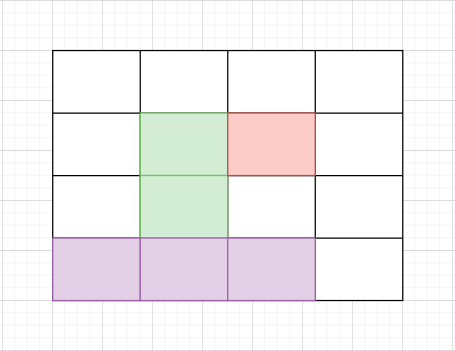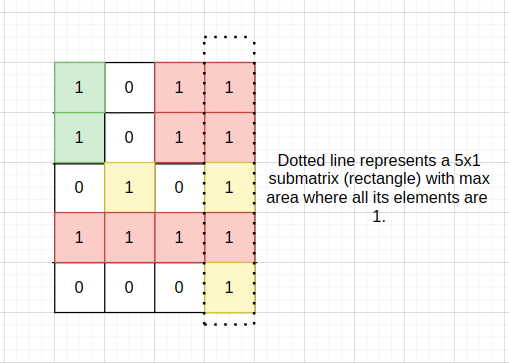0

# Maximum size rectangle sub-matrix with all 1's

Difficulty: EASY
Avg. time to solve
10 min
Success Rate
80%

Problem Statement
Suggest Edit

#### You are given an N*M size binary-valued matrix, where N is the number of rows and M is the number of columns. You need to return the size (area) of maximum size submatrix which consists of all 1’s i.e. the maximum area of a submatrix in which each cell has only the value ‘1’.``````In the above image, areas in green, red and violet colour are all submatrices of the original 4x4 matrix.
``````

#### Note:

``````1. Binary valued matrix has only two values in each cell : 0 and 1.
2. A submatrix is a matrix formed by selecting certain rows and columns from a larger matrix.
3. The area of a matrix with h rows and w columns is equal to h*w.
``````
##### Input Format:
``````The first line of the input contains an integer T denoting the number of test cases.

The first line of each test case contains two space-separated integers N and M, where N = number of rows in the given matrix and M = number of columns in the given matrix.

Then N lines follow for each test case:
Each line contains M space-separated integers(either 1 or 0).
``````
##### Output Format:
``````Print the area of maximum size submatrix of all 1’s in the given matrix.
``````
##### Note:
``````You do not need to print anything, it has already been taken care of. Just implement the given function.
``````
##### Constraints:
``````1 <= T <= 50
1 <= N,M <= 100

Time Limit: 1 sec
``````
##### Sample Input 1:
``````1
5 4
1 0 1 1
1 0 1 1
0 1 0 1
1 1 1 1
0 0 0 1
``````
##### Sample Output 1:
``````5
``````
##### Explanation for Sample Input 1:##### Sample Input 2:
``````1
4 4
1 1 1 1
1 1 1 1
0 0 1 1
0 0 1 1
``````
##### Sample Output 2:
``````8
``````Want to solve this problem? Login now to get access to solve the problems